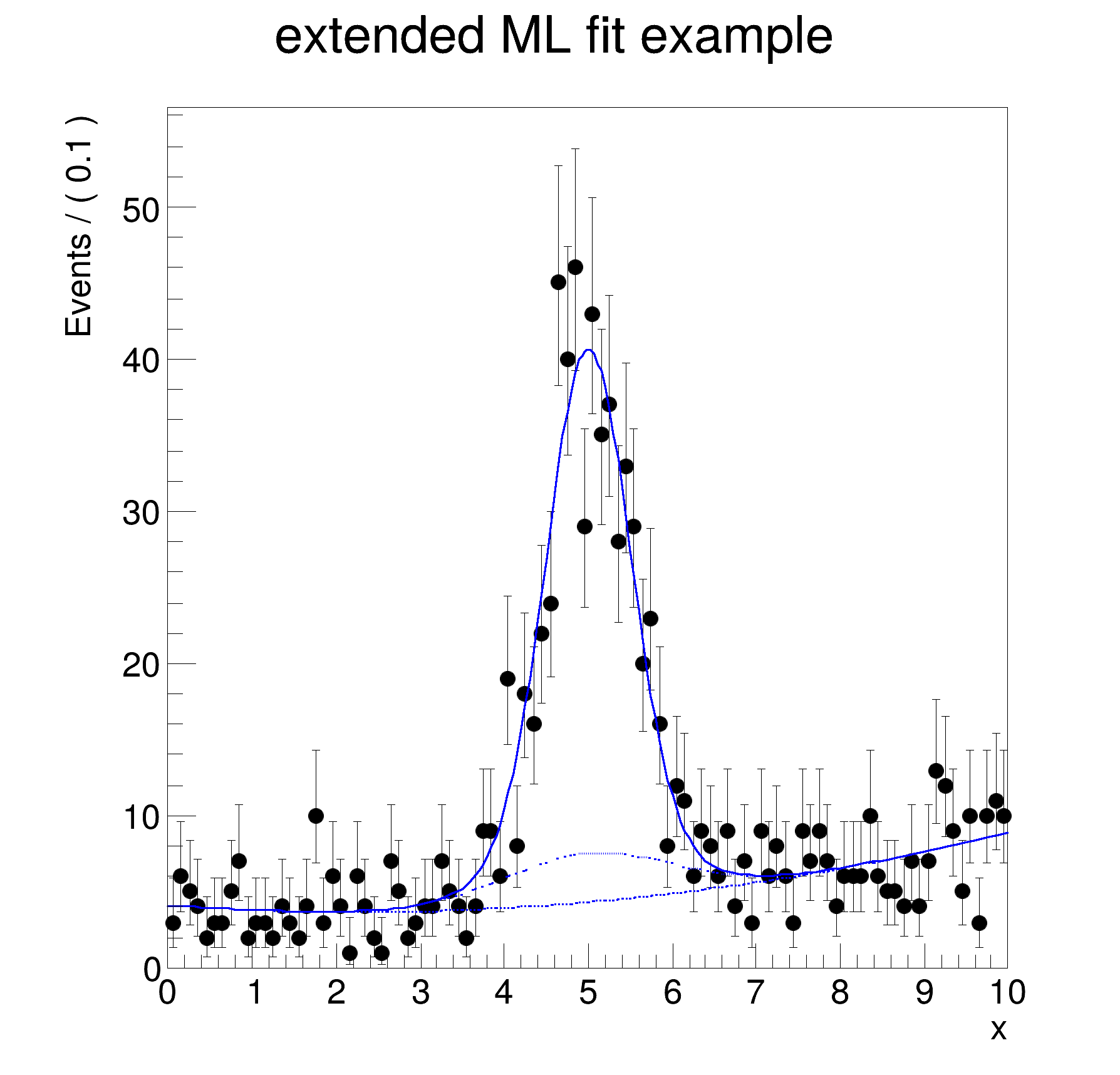ROOT   Reference Guide
Searching...
No Matches
rf202_extendedmlfit.C File Reference

## Detailed DescriptionSetting up an extended maximum likelihood fit.#include "RooRealVar.h"
#include "RooDataSet.h"
#include "RooGaussian.h"
#include "RooChebychev.h"
#include "RooExtendPdf.h"
#include "TCanvas.h"
#include "TAxis.h"
#include "RooPlot.h"
using namespace RooFit;
{
// S e t u p c o m p o n e n t p d f s
// ---------------------------------------
// Declare observable x
RooRealVar x("x", "x", 0, 10);
// Create two Gaussian PDFs g1(x,mean1,sigma) anf g2(x,mean2,sigma) and their parameters
RooRealVar mean("mean", "mean of gaussians", 5);
RooRealVar sigma1("sigma1", "width of gaussians", 0.5);
RooRealVar sigma2("sigma2", "width of gaussians", 1);
RooGaussian sig1("sig1", "Signal component 1", x, mean, sigma1);
RooGaussian sig2("sig2", "Signal component 2", x, mean, sigma2);
// Build Chebychev polynomial pdf
RooRealVar a0("a0", "a0", 0.5, 0., 1.);
RooRealVar a1("a1", "a1", 0.2, 0., 1.);
RooChebychev bkg("bkg", "Background", x, RooArgSet(a0, a1));
// Sum the signal components into a composite signal pdf
RooRealVar sig1frac("sig1frac", "fraction of component 1 in signal", 0.8, 0., 1.);
RooAddPdf sig("sig", "Signal", RooArgList(sig1, sig2), sig1frac);
//----------------
// M E T H O D 1
//================
// C o n s t r u c t e x t e n d e d c o m p o s i t e m o d e l
// -------------------------------------------------------------------
// Sum the composite signal and background into an extended pdf nsig*sig+nbkg*bkg
RooRealVar nsig("nsig", "number of signal events", 500, 0., 10000);
RooRealVar nbkg("nbkg", "number of background events", 500, 0, 10000);
RooAddPdf model("model", "(g1+g2)+a", RooArgList(bkg, sig), RooArgList(nbkg, nsig));
// S a m p l e , f i t a n d p l o t e x t e n d e d m o d e l
// ---------------------------------------------------------------------
// Generate a data sample of expected number events in x from model
// = model.expectedEvents() = nsig+nbkg
std::unique_ptr<RooDataSet> data{model.generate(x)};
// Fit model to data, extended ML term automatically included
model.fitTo(*data, PrintLevel(-1));
// Plot data and PDF overlaid, use expected number of events for pdf projection normalization
// rather than observed number of events (==data->numEntries())
RooPlot *xframe = x.frame(Title("extended ML fit example"));
data->plotOn(xframe);
model.plotOn(xframe, Normalization(1.0, RooAbsReal::RelativeExpected));
// Overlay the background component of model with a dashed line
model.plotOn(xframe, Components(bkg), LineStyle(kDashed), Normalization(1.0, RooAbsReal::RelativeExpected));
// Overlay the background+sig2 components of model with a dotted line
model.plotOn(xframe, Components(RooArgSet(bkg, sig2)), LineStyle(kDotted),
Normalization(1.0, RooAbsReal::RelativeExpected));
// Print structure of composite pdf
model.Print("t");
//----------------
// M E T H O D 2
//================
// C o n s t r u c t e x t e n d e d c o m p o n e n t s f i r s t
// ---------------------------------------------------------------------
// Associated nsig/nbkg as expected number of events with sig/bkg
RooExtendPdf esig("esig", "extended signal pdf", sig, nsig);
RooExtendPdf ebkg("ebkg", "extended background pdf", bkg, nbkg);
// S u m e x t e n d e d c o m p o n e n t s w i t h o u t c o e f s
// -------------------------------------------------------------------------
// Construct sum of two extended pdf (no coefficients required)
// Draw the frame on the canvas
new TCanvas("rf202_composite", "rf202_composite", 600, 600);
xframe->GetYaxis()->SetTitleOffset(1.4);
xframe->Draw();
}
@ kDashed
Definition TAttLine.h:48
@ kDotted
Definition TAttLine.h:48
Option_t Option_t TPoint TPoint const char GetTextMagnitude GetFillStyle GetLineColor GetLineWidth GetMarkerStyle GetTextAlign GetTextColor GetTextSize void data
RooAddPdf is an efficient implementation of a sum of PDFs of the form.
RooArgList is a container object that can hold multiple RooAbsArg objects.
Definition RooArgList.h:22
RooArgSet is a container object that can hold multiple RooAbsArg objects.
Definition RooArgSet.h:55
Chebychev polynomial p.d.f.
RooExtendPdf is a wrapper around an existing PDF that adds a parameteric extended likelihood term to ...
Plain Gaussian p.d.f.
Definition RooGaussian.h:24
A RooPlot is a plot frame and a container for graphics objects within that frame.
Definition RooPlot.h:43
static RooPlot * frame(const RooAbsRealLValue &var, double xmin, double xmax, Int_t nBins)
Create a new frame for a given variable in x.
Definition RooPlot.cxx:239
TAxis * GetYaxis() const
Definition RooPlot.cxx:1279
void Draw(Option_t *options=nullptr) override
Draw this plot and all of the elements it contains.
Definition RooPlot.cxx:652
RooRealVar represents a variable that can be changed from the outside.
Definition RooRealVar.h:37
virtual void SetTitleOffset(Float_t offset=1)
Set distance between the axis and the axis title.
Definition TAttAxis.cxx:298
The Canvas class.
Definition TCanvas.h:23
Double_t x[n]
Definition legend1.C:17
The namespace RooFit contains mostly switches that change the behaviour of functions of PDFs (or othe...
Definition Common.h:18
[#0] WARNING:InputArguments -- The parameter 'sigma1' with range [-inf, inf] of the RooGaussian 'sig1' exceeds the safe range of (0, inf). Advise to limit its range.
[#0] WARNING:InputArguments -- The parameter 'sigma2' with range [-inf, inf] of the RooGaussian 'sig2' exceeds the safe range of (0, inf). Advise to limit its range.
[#1] INFO:Minimization -- p.d.f. provides expected number of events, including extended term in likelihood.
[#1] INFO:Minimization -- RooAbsMinimizerFcn::setOptimizeConst: activating const optimization
[#1] INFO:Minimization -- The following expressions have been identified as constant and will be precalculated and cached: (sig1,sig2)
[#1] INFO:Minimization -- The following expressions will be evaluated in cache-and-track mode: (bkg)
[#1] INFO:Minimization -- RooAbsMinimizerFcn::setOptimizeConst: deactivating const optimization
[#1] INFO:Plotting -- RooAbsPdf::plotOn(model) directly selected PDF components: (bkg)
[#1] INFO:Plotting -- RooAbsPdf::plotOn(model) indirectly selected PDF components: ()
[#1] INFO:Plotting -- RooAbsPdf::plotOn(model) directly selected PDF components: (bkg,sig2)
[#1] INFO:Plotting -- RooAbsPdf::plotOn(model) indirectly selected PDF components: (sig)
0x7ffd780c2588/V- RooChebychev::bkg = 0.798919 [Auto,Dirty]
0x7ffd780c2ab8/V- RooRealVar::x = 5
0x7ffd780c06f8/V- RooRealVar::a0 = 0.441701 +/- 0.073185
0x7ffd780c0ae0/V- RooRealVar::a1 = 0.201081 +/- 0.117613
0x7ffd780c21a0/V- RooRealVar::nbkg = 504.206 +/- 39.3107
0x7ffd780bf478/V- RooGaussian::sig1 = 1 [Auto,Dirty]
0x7ffd780c2ab8/V- RooRealVar::x = 5
0x7ffd780c19d0/V- RooRealVar::mean = 5
0x7ffd780bff28/V- RooRealVar::sigma1 = 0.5
0x7ffd780c0ec8/V- RooRealVar::sig1frac = 0.837341 +/- 0.116841
0x7ffd780bf9d0/V- RooGaussian::sig2 = 1 [Auto,Dirty]
0x7ffd780c2ab8/V- RooRealVar::x = 5
0x7ffd780c19d0/V- RooRealVar::mean = 5
0x7ffd780c0310/V- RooRealVar::sigma2 = 1
0x7ffd780c1db8/V- RooRealVar::nsig = 495.799 +/- 39.2054
Date
July 2008

Definition in file rf202_extendedmlfit.C.